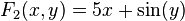### Introduction

In vector calculus, the Jacobian matrix is the matrix of all first-order partial derivatives of a vector-valued function. Specifically, supposeis a function which takes as input real n-tuples and produces as output real m-tuples. Such a function is given by m real-valued component functions,. The partial derivatives of all these functions with respect to the variables(if they exist) can be organised in an m-by-n matrix, the Jacobian matrix J of F, as follows:This matrix, whose entries are functions of, is also denoted byand.

(Note that some books define the Jacobian as the transpose of the matrix given above.)

The relation between Jacobian matrix and Gradient:The Jacobian matrix is important because if the function F is differentiable at a point p=(x1, ... , xn), which is a slightly stronger condition than merely requiring that all partial derivatives exist there, then the derivative of F at p is the linear transformationrepresented by the matrix. This linear transformation is the best linear approximation of the function F near the point p.

In the case m=n, the Jacobian matrix will be a square matrix, and its determinant, a function of x1, ... , xn, is the Jacobian determinant of F. It carries important information about the local behavior of F and can be thought of as a local expansion factor for volumes; it is used when performing variable substitutions in multi-variable integrals since it occurs prominently in the substitution rule for multiple variables.

### A Simple Example

Consider the functiongiven byThen we haveandand the Jacobian matrix of F isand the Jacobian determinant is### References & Resources

• http://en.wikipedia.org/wiki/Jacobian_matrix